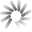## Fractalcoin# Fractalcoin Hashrate Chart

Warning: Fractalcoin is no longer being monitored as of 1-8-2016

The Fractalcoin hashrate chart provides the current FRAC hashrate right now as well as the history of Fractalcoin hashrate increases and descreases in graph format .

## FRAC Hashrate: 797.85 KH/sJan 08, 2016 10:15 AM UTC - 797,849 H/sLoading Fractalcoin Hashrate Chart

The Fractalcoin network hashrate chart can be used to visualize Fractalcoin mining hashrate increases and decreases viewable in segment options of daily, weekly, monthly, 3 months, 6 months, 1 year, 3 years, and all time.

## What is Fractalcoin Hashrate?

Fractalcoin hashrate is a calculated numerical value that specifies an estimate of how many hashes are being generated by Fractalcoin miners trying to solve the current Fractalcoin block or any given block.

Fractalcoin hashrate is represented in Hashes per Second or H/s.

The global Fractalcoin network hashrate is a calculated value and is measured in hashes per second (H/s). The calculation uses the current mining difficulty and the average Fractalcoin block time between mined blocks versus the defined block time as variables to determine the global Fractalcoin network hashrate.

As the Fractalcoin network hashrate goes up - the FRAC hashrate numbers get so large that abbreviations must be used.

The abbreviations are SI derived units representing the number of hashes performed in a one second time frame.

### Current Fractalcoin Hashrate

The current Fractalcoin hashrate is 797.85 KH/s, representing the global Fractalcoin network hashrate with a mining difficulty of 0.02 at block height 481,691. View the Fractalcoin hashrate chart for current and all time Fractalcoin historical hashrates.

Hashrate Unit/s Hash Hashes Per Second
H/s (Hash) 1 One
kH/s (KiloHash) 1,000 One Thousand
MH/s (MegaHash) 1,000,000 One Million
GH/s (GigaHash) 1,000,000,000 One Billion
TH/s (TeraHash) 1,000,000,000,000 One Trillion
EH/s (ExaHash) 1,000,000,000,000,000,000 One Quintillion
ZH/s (ZettaHash) 1,000,000,000,000,000,000,000 One Sextillion
YH/s (YottaHash) 1,000,000,000,000,000,000,000,000 One Septillion

It is important to point out the Fractalcoin hashrate does not determine how quickly or slowly each block is solved.

This timing, called the block time is enforced by the Fractalcoin mining difficulty value, which is adjusted upwards or downwards during each block difficulty retarget to keep blocks being solved at a constant time frame.

You can calculate Fractalcoin mining profits using the current FRAC hashrate difficulty and our Fractalcoin mining calculator.

## What is the Current Fractalcoin Hashrate?

The current Fractalcoin hashrate (FRAC hashrate) is 797.85 KH/s at block height 481,691 with a difficulty of 0.02.

Fractalcoin Hashrate Stats

### Current Fractalcoin Hashrate

Fractalcoin Global Hashrate
797.85 KH/s

### Fractalcoin Hashrate All Time High

Fractalcoin Hashrate on Oct 27, 2014 at block 126,136
4.44 GH/s

N/A
\$0.00 (0.00%)

24 hour change